I want to talk again today about the essential topic of analyzing statistical uncertainty – i.e., making error bars – but I want to frame the discussion in terms of a larger theme: our community’s often insufficiently critical adoption of elegant and sophisticated ideas. I discussed this issue a bit previously in the context of PMF calculations. To save you the trouble of reading on, the technical problem to be addressed is statistical uncertainty for high-variance data with small(ish) sample sizes.

Elegance and effectiveness are not the same thing. I should know something about it because I used to try to make a living in part by pursuing algorithmic elegance (‘resolution exchange’ simulation and path sampling for Jarzynski-based free energy calculations, to name two of my group’s less-than-world-changing developments). In other words, I’m both a perpetrator and victim of the problem.

Let’s dive into the bootstrap statistical analysis approach, which is truly beautiful and mathematically well-founded, but which can fail in at least one very important way – if we aren’t careful about using it in a manner consistent with its underlying assumptions. That’s an academic way of saying that I was trying to use bootstrapping ‘off the shelf’ without understanding it well. And in some sense, the problem I will discuss results from insufficient data even though the bootstrap is sometimes noted as useful for small samples … but also as potentially unreliable in that regime; see Schenker as well as Chernick & Labudde articles listed below.

First, what is the basic bootstrapping idea? It is a perfectly valid strategy for estimating statistical variation of arbitrary observables based on a given sample of data. The sample at hand (e.g., a set of configurations, or a set of energy values or rates) is assumed to be representative and hence is used as a proxy for the true distribution. From this proxy distribution, which is discrete even if the underlying space is continuous, numerous “bootstrap” resamples can be drawn, which are just samples with replacement. These bootstrap (re)samples represent a simulation of all possible samples assuming the initial sample is representative – and hence represent all variation consistent with that assumption.

With a set of bootstrap samples in hand, it is straightforward to make a confidence interval for an arbitrary observable. For each sample we have, we can calculate that observable and make a histogram. For example, if each sample consists of a single scalar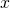, we could compute the “observable”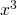for every sample and build a histogram of these. Likewise, from a set of configurations, we could build a histogram of energy values. Then, a 95% confidence interval would consist of the 2.5 and 97.5 %ile values from the histogram.

For later, remember this bootstrap confidence interval represents the variation expected if the original sample was a good proxy for the true distribution. This is a “frequentist” idea that we’ll revisit below. For now it sounds reasonable enough. And of course, bootstrapping is a very elegant procedure!

Let’s move on to the downside. We’ll consider a concrete, but very simple case where a failure can occur. Before you read on, or tell yourself a “toy” example is not important, I want to emphasize that our simple binomial example is highly relevant to certain types of real data, as I will describe later on in the post.

Assume we want to estimate the probabilityof the value 1 in a binomial distribution (where zero occurs with probability). That is,itself is the observable of interest. Say the sample we have in hand to estimateis (1,0,0,0,0). Of course we would estimate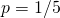. But how confident are we based on just five draws from the binomial distribution? Perhapsis actually much smaller and we were lucky to see a single 1. Or perhapswas larger and we got unlucky. How do we think about this?

The bootstrapping procedure readily provides a confidence interval, as described above … but we will see that it is seriously flawed. Bootstrapping calls for sampling with replacement repeatedly from the original (1,0,0,0,0) sample. Sampling with replacement means that we draw sets of five elements from the original set and every element is chosen with equal probability. Thus, any element may randomly occur more than once (which is probably easier to think about if we had five different values, but we’ll stick to binomial). In our binomial case, a couple of the (re)samples might look like (0,1,0,0,1) or (0,0,0,0,0). Imagine drawing thousands of such samples and estimatingfrom each, simply based on the fraction of ones – i.e., 2/5 or 0 for the preceding examples. Then we can construct a histogram of these values and use the 2.5 and 97.5%iles to make a confidence interval. This interval represents the range of the 95% most likely values if our original sample was representative of the true distribution.

For the binomial case, there are few enough possibilities that we can easily predict what will happen in a bootstrap process. In particular, we know that in generating each bootstrap sample we have a 1/5 chance of a 1 and 4/5 chance of 0. Let’s focus on a key occurrence, the outcome (0,0,0,0,0), which leads to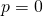and has a probability of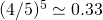. This tells us right away that the lower limit of the confidence interval will be 0 (because the 2.5% is less than 33% and hence occurs atin the histogram). In fact, sinceoccupies 33% of the probability, even when we chop off the lowest 2.5%, bootstrapping’s confidence interval still covers for 30% of its 95%!

And there we have the problem: the bootstrapping confidence interval wrongly indicates that there’s a decent chance that. Why is that wrong? Well, our sample of just five binomial values already has a 1 in it. Although we don’t have much data, we do have enough data to rule out certain models:must be finite and not zero. We can use Bayesian ideas to quantify our common sense, but let’s hold off on that for a moment.

Let me now explain why a binomial example has been relevant for my own research on reasonably complex biomolecular systems. We have been simulating the folding of proteins using the “weighted ensemble” (WE) path sampling method, which can yield unbiased rate estimates. Each (quite expensive) WE run yields an estimate for the folding rate, but there is a very large variance among these estimates: one run may yield a rate that is several orders of magnitude larger than another. And a set of 10-30 WE folding runs yields a set of rates whose average is dominated by a small number (< 5) of the runs. The small number of large values is essentially like the 1’s in a binomial distribution, with the remainder like the 0’s. In that sense it’s the same situation, and bootstrapping will suggest an artifactually small lower limit for the confidence interval of the folding rate. So for me, the binomial example is very relevant. (Note that a “regular” confidence interval based on the standard error of the mean will yield unphysical negative values because the data is highly non-Gaussian. Note also that one difference between the folding data and binomial samples is that in the binomial case we know that 1 is the largest possible value whereas we don’t know the largest possible value for the folding rate ahead of time. However, the critique of the lower confidence limit is still valid.)

We’ve seen what goes wrong procedurally with the bootstrap in for a high-variance small sample, but what goes wrong theoretically? Here we start to get into issues of frequentism and Bayesianism, but I want to avoid jargon and abstraction. Let’s just remind ourselves what the bootstrap procedure does. It generates a rather complete distribution of outcomes assuming that the sample in hand is a good approximation to the true underlying distribution. But in generating a confidence interval for, say, theparameter of a binomial distribution, I would argue it’s clear that it’s not really the possible outcomes we care about. Rather, we want to estimate the likelihood that a givencould have generated the sample we have, which FYI is the Bayesian point of view: find the models most consistent with data. Also, as with many statistical approaches, bootstrapping seems to be theoretically founded on large-numbers statistics, which clearly are lacking in our example.

More concretely, given the binomial sample (1,0,0,0,0), it’s clear this is consistent with, but what about other values of? This is easy to quantify in the binomial case because from elementary binomial statistics, the probability of a sample with a single 1 is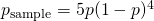. From a Bayesian perspective, we can use this expression as a basis for estimating the probability that our sample came from any. That is, even though strictly speaking the expression for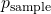gives the fraction of five-element samples expected to have a single 1 for a fixed value of, we can reverse the direction of the logic and useas our best guess for the probability (density) that our particular sample came from any given value of. So aside from the issue of normalization, we can think of it as a function of:. Notice that this function has a maximum atand it correctly tells us there is zero probability thator, in sharp contrast to bootstrapping.

That’s where we have to end this discussion. I don’t have the time, space, or expertise to give a complete description of the best way to proceed with small-sample data of a binomial character. (I think a partial solution involves ‘Bayesian bootstrapping’ – see our initial efforts here.) But I hope you have understood the basic reasons why even a beautiful idea like bootstrapping can go wrong. Of course, it’s fair to say that I was guilty of trying to use bootstrapping without understanding it thoroughly enough. In any case, I hope my experience will be useful for you.

In short, question authority, question precedent! Examine the assumptions underlying the calculations you do.